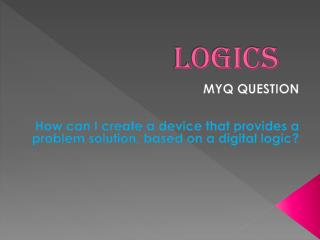DownloadDownload PresentationLOGICS

# LOGICS

Télécharger la présentation## LOGICS

- - - - - - - - - - - - - - - - - - - - - - - - - - - E N D - - - - - - - - - - - - - - - - - - - - - - - - - - -
##### Presentation Transcript

1. LOGICS MYQ QUESTION How can I create a device that provides a problem solution, based on a digital logic?

2. PLAN INVESTIGATION • All the information about the investigation stage should be placed in a blog or wiki that has to be created by the student. It should be published and shared with the Teacher • Define following terms: Digital logic, Logic gate, Truth table, Integrated Circuit, Led, Breadboard, Breadboard jumpers. Every “term definition” should be illustrated by placing two pictures • Compile the basic logic gates symbols • Sketch the true tables for the logic gates investigated before • Learn how to use a specific software (Logic Circuit Designer, Atanua) to create digital circuits based on digital logic

3. Definitions of several words:

4. The digital logic: • 0 = false = no 1 = true = yes • The digital logic or the binary logic is the system of the computers and it consists of two values numbers: 0 and 1. So 0 means false and 1 means true. Using this two-valued logic system, every statement or condition must be either "true" or "false. And the 1 mean close and is when the current CAN pass and 0 means open and current CANT passes by the circuit.

5. DIGITAL LOGIC

6. LOGIC GATE: • There are seven basic logic gates: AND, OR, NOT, NAND and NOR. • So the logic gate AND means that all the inputs need to be true (1) for the output (result) to be true otherwise it will be false. • The OR logic gate means that all the inputs need to be false for the output to be false otherwise it will be true the output. • The NOT gate means the opposite of the input, for example If one of the input were false (0) the opposite will be true (1). • The NAND is an AND gate with a NOT gate. It is the opposite of AND gate. For example if the result of a AND gate is true in the NAND gate it will be false. • Finally the NOR logic gate means is an OR gate with a NOT gate. This gate is the opposite of the OR gate, the result will be the opposite. If the result of OR gate is false in the NOR gate it will be true.

7. LOGIC GATES

8. True table • A truth table is a mathematical table used in logic. Practically, a truth table contains one column for each input variable (for example, A and B), and one final column for all of the possible results of the logical operation (for example, A XOR B).Each input must have a 1(true) or 0(false) value.

9. True table

10. An integrated circuit • Sometimes called a chip or microchip, are electronic circuits where all the components (transistors, diodes, resistors and capacitors) has been put into the surface of a thin substrate of semiconductor material. This Integrated circuits can be found in almost every modern electrical device; such as computers, cars, television etc.

11. AN INTEGRATED CIRCUIT

12. LED • Is a little ball use in electronic devices

13. Breadboard: • A breadboard is used to build and test circuits quickly before finalizing the circuit. The breadboard has many holes in it, in which circuit components like ICs andresistors can be inserted.